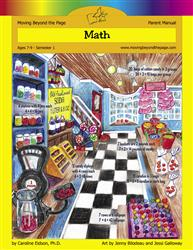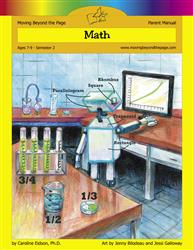# Common Core Alignment

CCSS.Math.Content.3.OA.7 - Fluently multiply and divide within 100, using strategies such as the relationship between multiplication and division (e.g., knowing that 8 × 5 = 40, one knows 40 ÷ 5 = 8) or properties of operations. By the end of Grade 3, know from memory all products of two one-digit numbers.

## 5: MathUnit 1: Multiplication and Division I
Lesson 10: More Practice With 2, 3, 4, and 5
Lesson 11: Multiples of 10
Lesson 12: Problem Solving With Multiplication
Lesson 15: Unit Test
Lesson 7: Multiples of 2 and 3
Lesson 8: More Practice With 2 and 3
Lesson 9: Multiples of 4 and 5
Final Project: Multiples Mini-PostersUnit 4: Multiplication and Division II
Lesson 1: Mastering More Multiplication Facts
Lesson 12: Unit Test
Lesson 2: Multiples of 6 and 7
Lesson 3: Multiples of 8 and 9
Lesson 4: Practicing All of the Multiples
Lesson 5: Associative Property of Multiplication
Lesson 6: Distributive Property of Multiplication
Lesson 7: Reviewing Properties of Multiplication
Lesson 8: Revisiting Division
Lesson 9: Multiplication and Division Facts PracticeUnit 9: Skills Review
Lesson 1: Reviewing Multiplication and Division